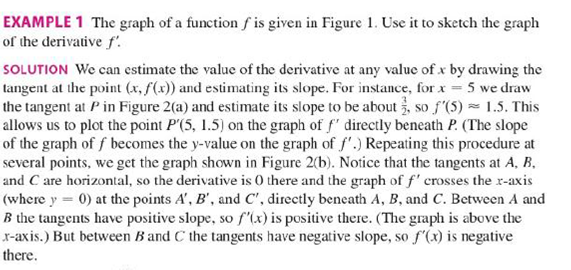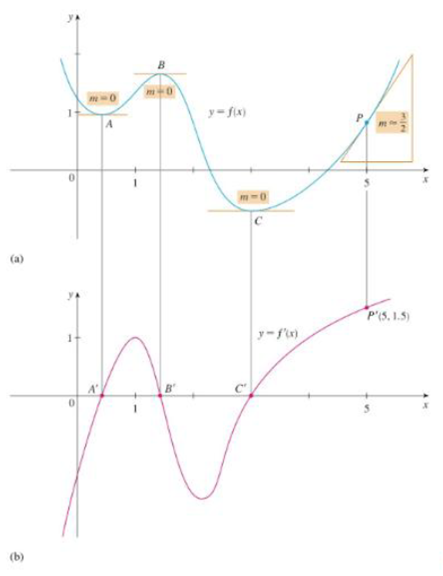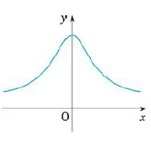Chapter 2.8, Problem 6E

Chapter
Section
Textbook Problem

Trace or copy the graph of the given function .f. (Assume that the axes have equal scales.) Then use the method of Example 1 to sketch the graph of f' below it.Example 1FIGURE 1FIGURE 2To determine

To Sketch: The graph of f below the graph of f.

Explanation

From the given graph, it is observed that the graph of f contains the horizontal tangent at one point. Let this point be A.

Note that, the value of the derivative will be zero at the point where the function has the horizontal tangent.

Thus, the graph of f will be zero at the point A.

From the point A to left, the slope of the graph f is strictly positive which implies that the derivative graph f  must have a functional value in positive

Still sussing out bartleby?

Check out a sample textbook solution.

See a sample solution

The Solution to Your Study Problems

Bartleby provides explanations to thousands of textbook problems written by our experts, many with advanced degrees!

Get Started

Describe the set of points (x,y) such that x2+y2=0.

Finite Mathematics and Applied Calculus (MindTap Course List)

In Exercises 1124, find the indicated limits, if they exist. 15. limx2x+3x29

Applied Calculus for the Managerial, Life, and Social Sciences: A Brief Approach

Divide the following fractions and reduce to lowest terms. 26.

Contemporary Mathematics for Business & Consumers

In Problems 1-8, use a calculator to evaluate each expression. 7.

Mathematical Applications for the Management, Life, and Social Sciences

The slope of the tangent line at the point where to the curve y = sin 2t, x = cos 3t is: 3 −3

Study Guide for Stewart's Single Variable Calculus: Early Transcendentals, 8th